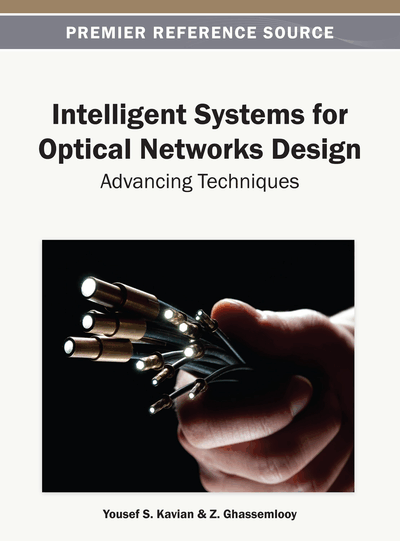# Artificial Bee Colony Approach for Routing and Wavelength Assignment in Optical WDM Networks

Goran Z. Markovic (University of Belgrade, Serbia)
DOI: 10.4018/978-1-4666-3652-1.ch008
Available
\$37.50
No Current Special Offers

## Abstract

Routing and Wavelength Assignment (RWA) of lightpaths in optical WDM networks is a challenging task that belongs to a class of complex combinatorial problems. To solve the RWA problem of realistic size, heuristic or meta-heuristic approaches have to be used. In this chapter, an artificial bee colony metaheuristic approach, known as the Bee Colony Optimization (BCO), is used to solve the RWA problem for static lightpath establishment in wavelength routed optical WDM networks. The BCO metaheuristic is tailored here to solve the Max-RWA problem in which the objective is to maximize the number of established lightpaths for a given number of wavelengths. Behind a comprehensive description of the proposed BCO-RWA algorithm, the numerical results obtained by numerous simulations performed over widely used real world European Optical Network (EON) topology are given and compared with some other approaches used to solve the same problem.
Chapter Preview
Top

## Introduction

Wavelength Routed Optical Networks (WRONs) employing Wavelength Division Multiplexing (WDM) technique are considered as the most promising solution to satisfy the huge amount of traffic that is expected in next generation communication networks. Routing and Wavelength Assignment (RWA) of lightpaths is a central problem that has to be solved in WRON design and operation. A key matter concerned with routing of lightpaths is that no two lightpaths established over a fiber link can share the same wavelength. Additionally, if there are no wavelength converters in network, a lightpath has to be assigned the same wavelength on all links through which it traverses. Extended review of the RWA problem in optical WDM networks could be found in (Murthy and Gurusamy, 2002).

We study here the static case of the RWA problem in which the entire set of lightpath requests that need to be setup over the network is completely known in advance. It is also known as the Static Lightpath Establishing (SLE) problem. SLE is a combinatorial optimization problem that could be formulated as Mixed Integer Linear Program (MILP) and solved in off-line manner. However, it has been shown that static RWA is NP (Nondeterministic-polynomial time) complete problem that could be solved only in the case of small problem dimensionalities. For larger size problems, various heuristics or meta-heuristic algorithms have been proposed. Metaheuristics are general high level procedures that coordinate simple heuristics and rules to find approximate solutions of computationally difficult combinatorial problems. Since approximate algorithms explore only a fraction of the solution space associated to a problem instance, they are able to produce solutions within a reasonable computation time, but without necessarily providing guarantees of solution quality. Therefore, such schemes are suitable in environments where a solution is required in a short time compared to mathematical schemes like ILP or graph colouring. Each heuristic technique has its own advantages and disadvantages and the efficiency of applying a particular technique depends on many factors like the computational complexity, execution time, quality of solution, robustness and so forth. Researchers have developed various global optimization metaheuristic algorithms such as the Simulated Annealing (SA), Tabu Search (TS), and different Evolution Algorithms (EA).

In recent years, a new class of metaheuristics, known as the Swarm Intelligence (SI), have particularly attracted the significant interest of many researchers to solve various complex optimization problems. SI is an emerging optimization approach that takes inspiration from the collective behaviour of social colonies of insects or other animal societies, such as colonies of ants, bees, termites, flocks of birds, schools of fish and others in order to develop some artificial algorithms which can mimic insect's problem solution abilities. Inspired by natural behavior of various social colonies, researchers have developed intelligent search algorithms by modelling interactions in natural swarms.

Our focus here is on the bee swarms. Studies on honey bees are in an increasing trend in the literature during the last few years. A number of artificial algorithms inspired by natural bees’ activities have been proposed to solve different optimization problems. In this chapter, a novel artificial bee algorithm, based on Bee Colony Optimization (BCO) metaheuristic is proposed to solve the static RWA problem in WRON networks. The BCO is particularly tailored to solve the Max-RWA problem in which the objective is to maximize the number of established lightpaths for a given number of wavelengths in optical WDM networks operating under wavelength continuity constraint (Marković, 2007). To the best of our knowledge, the BCO-RWA is the first artificial bee algorithm proposed in literature to solve the RWA problem in optical WDM networks (Marković et al, 2007). Recently, Rubio-Largo et al. (2011) used the Artificial Bee Colony (ABC) algorithm to solve the static RWA problem as a multi-objective optimization problem. In addition, Rashedi et al. (2011) applied the ABC algorithm to solve dynamic RWA problem for minimizing the blocking probability in optical WDM networks.

## Complete Chapter List

Search this Book:
Reset